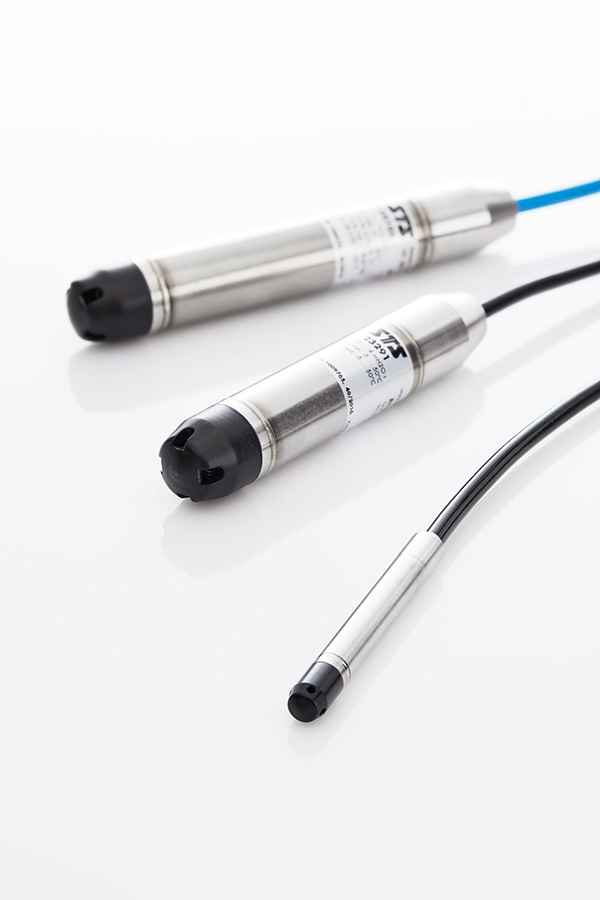p(h) = ρ * g * h + p0

p(h) = 静水压力
ρ = 液体密度
g = 重力
h =液柱高度Figure 1: Examples of level sensors for hydrostatic pressure measurement